# Class 12 Maths NCERT Solutions for Chapter 9 Differential Equations Exercise 9.3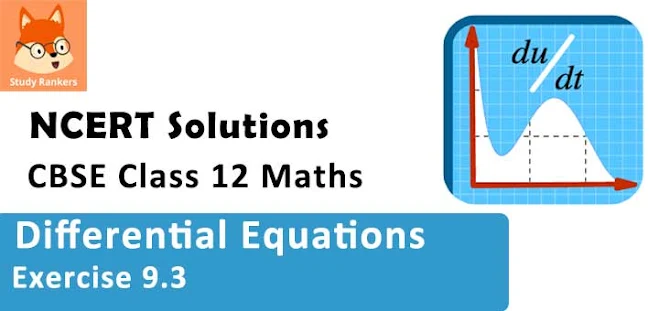### Differential Equations Exercise 9.3 Solutions

1. Form a differential equation representing the given family of curves by eliminating arbitrary constants a and b.
x/a + y/b = 1

Solution

x/a + y/b = 1
Differentiating both sides of the given equation with respect to x, we get :
1/a + (1/b)(dy/dx) = 0
⇒ 1/a + (1/b)y' = 0
Again , differentiating both sides with respect to x, we get :
0 + (1/b)y" = 0
⇒ (1/b)y" = 0
⇒ y" = 0
Hence, the required differential equation of the given curve is y" = 0.

2. Form a differential equation representing the given family of curves by eliminating arbitrary constants a and b.
y2 = a (b2 – x2)

Solution

y2 = a (b2 – x2)
Differentiating both sides with respect to x, we get: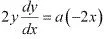⇒ 2yy' = -2ax
⇒ yy' = -ax ...(1)
Again, differentiating both sides with respect to x, we get :
y'. y' + yy" = -a
⇒ (y')2 + yy" = -a ...(2)
Dividing equation (2) by equation (1), we get :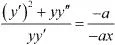⇒ xyy" + x(y')2 - yy" = 0
This is the required differential equation of the given curve.

3. Form a differential equation representing the given family of curves by eliminating arbitrary constants a and b.
y = a e3x + b e2x

Solution

y' = 3ae3x - 2be-2x ...(2)
Again, differentiating both sides with respect to x, we get :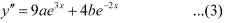Multiplying equation (1) with 2 and then adding it to equation (2) , we get :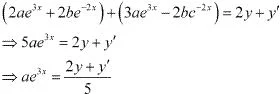Now, multiplying equation (1) with 3 and subtracting equation (2) from it, we get :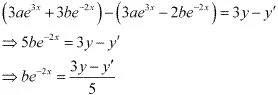Substituting the values of ae3x and be-2x in equation (3), we get :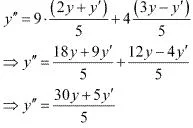⇒ y'' = 6y + y'
⇒ y'' - y' - 6y = 0
This is the required differential equation of the given curve.

4. Form a differential equation representing the given family of curves by eliminating arbitrary constants a and b.
y = e2x (a + bx)

Solution

y = e2x (a + bx)
Differentiating both sides with respect to x, we get :
y' = 2e2x (a + bx) + e2x.b
⇒ y' = e2x (2a + 2bx + b)  ...(2)
Multiplying equation (1) with 2 and then subtracting it from equation (2),we get :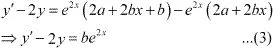Differentiating both sides with respect to x, we get :
y" - 2y' = 2be2x ...(4)
Dividing  equation (4) by equation (3), we get :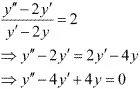This is the required differential equation of the given curve.

5. Form a differential equation representing the given family of curves by eliminating arbitrary constants a and b.
y = ex (a cos x + b sin x)

Solution

y = ex (a cos x + b sin x)
Differentiating both sides with respect to x, we get :
y' = ex (a cos x + b sin x) + ex (- a sin x + b cos x)
= y' = ex [(a + b) cos x - (a - b) sin x] ...(2)
Again, differentiating with respect to x, we get :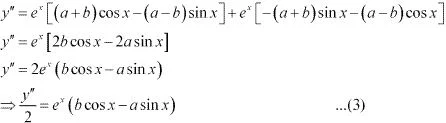Adding equations (1) and (3) , we get :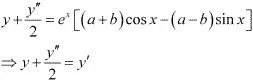⇒ 2y + y" = 2y'
⇒ y" - 2y' + 2y = 0
This is the required differential equation of the given curve.

6. Form the differential equation of the family of circles touching the y-axis at the origin.

Solution

The centre of the circle touching the y - axis at origin lies on the x - axis.
Let (a, 0) be the centre of the circle.
Since it touches the y - axis at origin, its radius is a.
now, the equation of the circle with centre (a, 0) and radius (a) is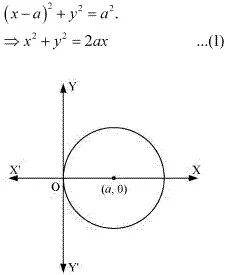Differentiating equation (1) with respect to x, we get :
2x + 2yy' = 2a
⇒ x + yy' = a
Now, on substituting the value of a in equation (1), we get :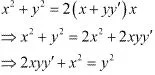This is the required differential equation.

7. Form the differential equation of the family of parabolas having vertex at origin and axis along positive y-axis.

Solution

The equation of the parabola having the vertex at origin and the axis along the positive y axis is :
x2 = 4ay ...(1)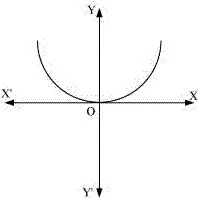Differentiating equation (1) with respect to x, we get :
2x = 4ay'  ...(2)
Dividing equation (2) by equation (1), we get :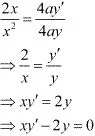This is the required differential equation.

8. Form the differential equation of the family of ellipses having foci on y-axis and centre at origin.

Solution

The equation of the family of ellipses having foci on the y - axis and the centre at origin is as follows:
x2 /b2  + y2 /a2  = 1 ...(1)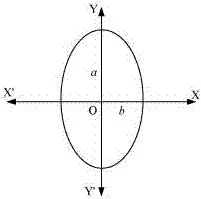Differentiating equation (1) with respect to x, we get :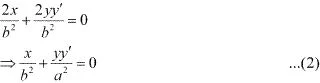Again, differentiating with respect to x, we get :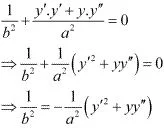Substituting this value in equation (2), we get :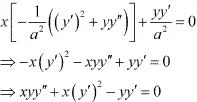This is the required differential equation.

9. Form the differential equation of the family of hyperbolas having foci on x-axis and centre at origin.

Solution

The equation of the family of hyperbolas with the centre at origin and foci along the x - axis is :
x2 /a2  + y2 /b2  = 1 ...(1)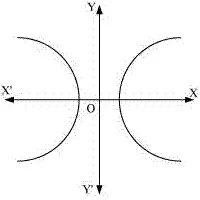Differentiating both sides of  equation (1) with respet to x, we get :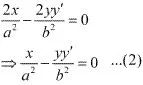Again, differentiating both sides with respect to x, we get :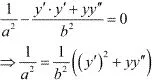Substituting the value of 1/a2 in equation (2), we get :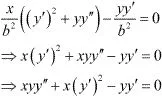This is the required differential equation.

10. Form the differential equation of the family of circles having centre on y-axis and radius 3 units.

Solution

Let the centre of the circle on y - axis be (0, b).
The differential equation of the family of circles with centre at (0, b) and radius 3 is as follows :
x2 + (y - b)2 = 32
⇒ x2 + (y - b)2 = 9 ...(1)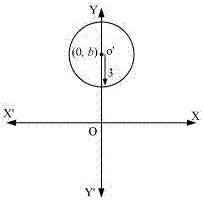Differentiating equation (1) with respect to x, we get :
2x + 2(y - b). y' = 0
⇒ (y - b). y' = -x
⇒ y - b = -x/y'
Substituting the value of (y - b) in equation (1), we get :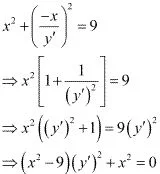This is the required differential equation.

11. Which of the following differential equations has y = c1 ex + c2 e–x as the general solution ?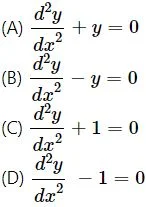Solution
The given equation is :
y = c1 ex + c2 e–x ...(1)
Differentiating with respect to x, we get :
dy/dx = c1ex - c2e–x
Again, differentiating with respect to x, we get :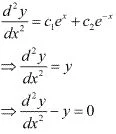This is the required differential equation of the given equation of curve.
Hence, the correct answer is B.

12. Which of the following differential equation has y = x as one of its particular solution ?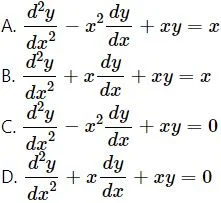Solution
The given equation of curve is y = x.
Differentiating with respect to x, we get :
dy/dx =1 ...(1)
Again, differentiating with respect to x, we get :
d2 y/dx2 = 0 ...(2)
Now, on substituting the values of y, d2 y/dx2, and dy/dx from equation (1) and (2) in each of the given alternatives, we find that only the differential equation given in alternative C is correct.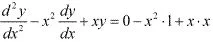= -x2 + x2
= 0
Hence, the correct answer is C.